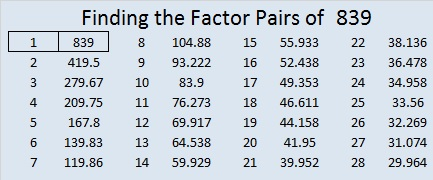# 839 and Level 5

839 is the sum of the five prime numbers from 157 to 179:

• 157 + 163 + 167 + 173 + 179 = 839Print the puzzles or type the solution on this excel file: 10-factors-835-842

• 839 is a prime number.
• Prime factorization: 839 is prime.
• The exponent of prime number 839 is 1. Adding 1 to that exponent we get (1 + 1) = 2. Therefore 839 has exactly 2 factors.
• Factors of 839: 1, 839
• Factor pairs: 839 = 1 × 839
• 839 has no square factors that allow its square root to be simplified. √839 ≈ 28.9654967

How do we know that 839 is a prime number? If 839 were not a prime number, then it would be divisible by at least one prime number less than or equal to √839 ≈ 28.97. Since 839 cannot be divided evenly by 2, 3, 5, 7, 11, 13, 17, 19, or 23, we know that 839 is a prime number.This site uses Akismet to reduce spam. Learn how your comment data is processed.